# Fractions + functions - math problems

#### Number of problems found: 275

• Cancel fractionsCompress the expression of factorial: (n+6)!/(n+4)!-n!/(n-2)!
• Cake fractions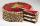Thomas ate 1/3 of cake, Bohouš of the rest of the cake ate 2/5. What fraction of cake left over for others?
• Equation - inverseSolve for x: 7: x = 14: 1000
• One sixth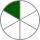How many sixths are two thirds?
• ExpressionIf it is true that ? is:
• Ratio 11Simplify this ratio 10 : 1/4
• Reciprocal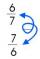Forgiven real numbers, calculate the reciprocal numbers.
• Tree trunk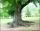Calculate the average tree trunk with a circumference of 149 cm.
• Mixed with percentagesCalculate 33 1/3% of 570.
• Wage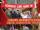Increasing the minimum wage while maintaining the other parameters of the system, unemployment will be:
• Proportional relationship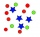The ordered pairs (6,24) and (1, s) represent a proportional relationship. Find the value of s.
• Ratio - proportion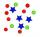Reduce the number 16 in proportion 3:2 5:4 11:8
• Insert 6Insert four harmonic means between 3/7 and 3/19
• Percentages 5\$5.25 is 7 1/2% of what number?
• Lunch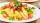Jane eats whole lunch for the 30 minutes. Which part of the lunch is eaten in 180 seconds?
• SupermarketIn a local supermarket, 3/5 kilograms of squid cost 156.00. How do 4 kilograms of squid cost?
• AndreAndre, Thomas, and Ivan split 88 postage stamps in a 2:5:4 ratio. How much did Thomas get?
• Sales taxA sales tax on a Php 10,800 appliance is Php.1,620. What is the rate of sales tax?
• Ratio of countsThere are 15 boys and 13 girls in the class. What are the ratio of boys and girls?
• Percent calculationCalculate 51% if 99% is 79.

Do you have an interesting mathematical word problem that you can't solve it? Submit a math problem, and we can try to solve it.

We will send a solution to your e-mail address. Solved examples are also published here. Please enter the e-mail correctly and check whether you don't have a full mailbox.

Please do not submit problems from current active competitions such as Mathematical Olympiad, correspondence seminars etc...

Need help calculate sum, simplify or multiply fractions? Try our fraction calculator.